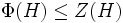# Critical subgroup

This article is about a definition in group theory that is standard among the group theory community (or sub-community that dabbles in such things) but is not very basic or common for people outside.
VIEW RELATED: Analogues of this | Variations of this | Opposites of this |
View a list of other standard non-basic definitions

WARNING: POTENTIAL TERMINOLOGICAL CONFUSION: Please don't confuse this with critical group

## Definition

### Symbol-free definition

A subgroup of a group of prime power order (or more generally, of a possibly infinite p-group) is termed a critical subgroup if it is characteristic in the whole group and satisfies the following three conditions:

1. The subgroup is a Frattini-in-center group: Its Frattini subgroup is contained in its center.
2. The subgroup is a commutator-in-center subgroup: Its commutator with the whole group is contained in its center.
3. The subgroup is a self-centralizing subgroup: Its centralizer in the whole group is contained in it.

### Definition with symbols

Let$G$ be a group of prime power order (or more generally, a possibly infinite p-group).

A subgroup$H$ of$G$ is said to be critical if it is characteristic in$G$, and the following three conditions hold:

1.$\Phi(H) \le Z(H)$, i.e., the Frattini subgroup is contained inside the center (i.e.,$H$ is a Frattini-in-center group).
2.$[G,H] \le Z(H)$ (i.e.,$H$ is a commutator-in-center subgroup of$G$).
3.$C_G(H)= Z(H)$ (i.e.,$H$ is a self-centralizing subgroup of$G$).

## Relation with other properties

### Group properties satisfied

Any critical subgroup satisfies the following group properties:

## Facts

1. Every group of prime power order has a critical subgroup. Further information: Thompson's critical subgroup theorem, Analysis of Thompson's critical subgroup theorem
2. More generally, every infinite p-group that is also abelian-by-nilpotent has a critical subgroup. Further information: Analogue of critical subgroup theorem for infinite abelian-by-nilpotent p-group
3. A group of prime power order can arise as a critical subgroup of some group, if and only if it is a Frattini-in-center group. Further, any Frattini-in-center group is a critical subgroup of itself.
4. A critical subgroup that is also extraspecial as a group must equal the whole group. For full proof, refer: extraspecial and critical implies whole group
5. Abelian Frattini subgroup implies centralizer is critical

## Metaproperties

### Left realization

A group of prime power order can arise as a critical subgroup of some group, if and only if it is a Frattini-in-center group (in other words, its Frattini subgroup is contained in its center). Further, any Frattini-in-center group is a critical subgroup of itself.

### Right realization

By Thompson's critical subgroup theorem, every group of prime power order possesses a critical subgroup.